Learning Library

# Decimal Dash: Practice Ordering Decimals

Riddle me this, MathMan! Your kid can use his math skills to answer the word puzzle at the bottom of each page.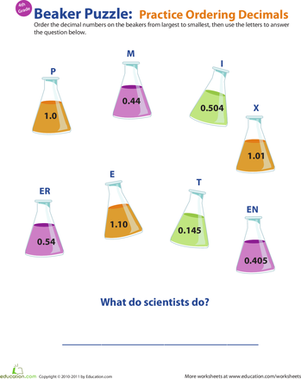### Ordering Decimals Practice

Does your fourth grader need help with ordering decimals? Bolster his decimal understanding with this beaker-booked worksheet.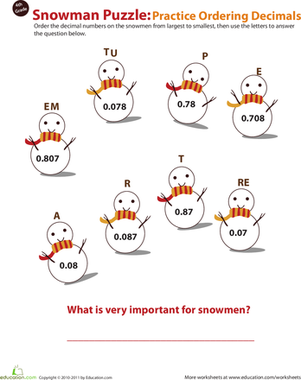### Ordering Decimal Numbers

Give your fourth grader's math skills a hand with our decimal worksheet. Snowmen keep him company as he practices ordering decimal numbers from big to small.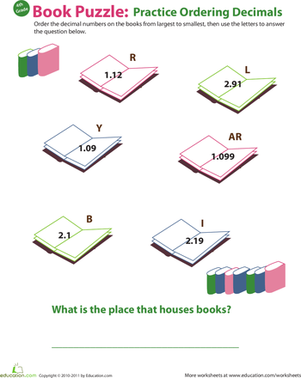### Order Decimals from Greatest to Least

Encourage your fourth grader to dive into decimals with this bookish math worksheet. In this worksheet he'll order decimals from greatest to least.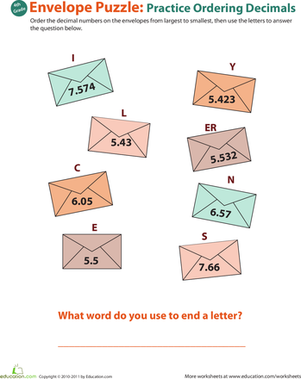### Decimal Dash: Practice Ordering Decimals #4

Packed with decimal practice, this worksheet will boost your kid's understanding of this math concept. Put decimal numbers in order from biggest to smallest.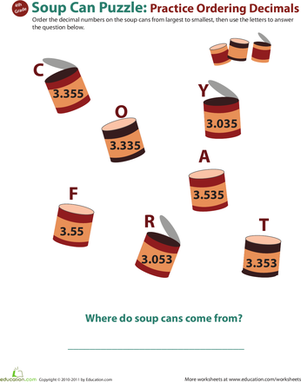### Decimal Dash: Practice Ordering Decimals #5

Decimals can be confusing for kids, but a little practice is all they need to understand this important fourth grade concept. Order soup cans from big to small.### Decimal Dash: Practice Ordering Decimals #6

Is your fourth grader struggling to wrap her head around decimals? Don't despair: offer her a little practice, and she'll have this concept down in no time.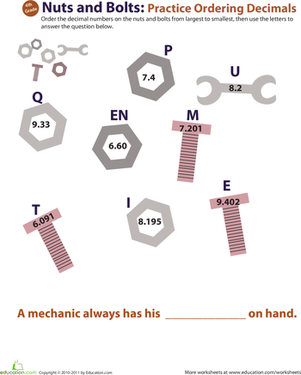### Decimal Dash: Practice Ordering Decimals #7

Decimals can be tricky, but don't let your fourth grader bolt: encourage him to learn about the not-quite-whole numbers, and he'll be decimal savvy in no time.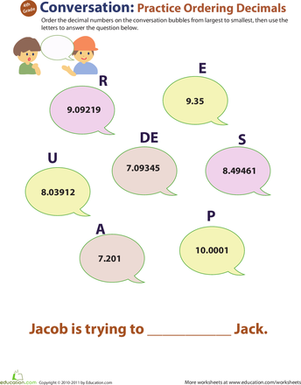### Decimal Dash: Practice Ordering Decimals #8

Help your fourth grader get a leg up on decimals with this colorful math worksheet. She'll practice putting decimal numbers in order from largest to smallest.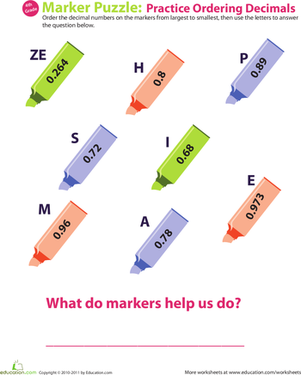### Decimal Dash: Practice Ordering Decimals #9

Is your fourth grader confused about decimals? Give his decimal understanding a boost with this fun worksheet that asks him to put decimal numbers in order.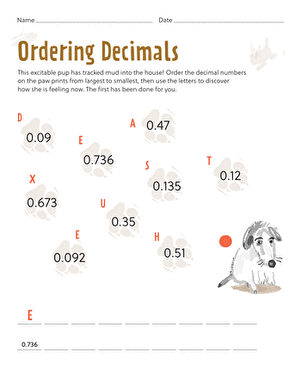### Order Decimals

Your child will order decimals by putting paw prints in order from big to small. He'll rank numbers and gain an understanding of this fourth grade concept.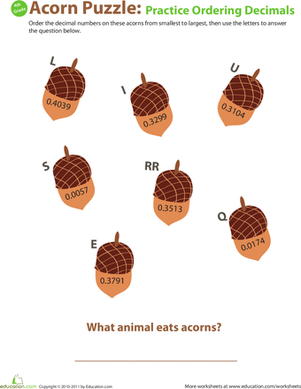### Putting Decimals in Order

Encourage her to get to know these special numbers better with some practice. This acorn-filled worksheet gets her putting decimals in order from big to small.

Create new collection

0

### New Collection>

0 items

What could we do to improve Education.com?